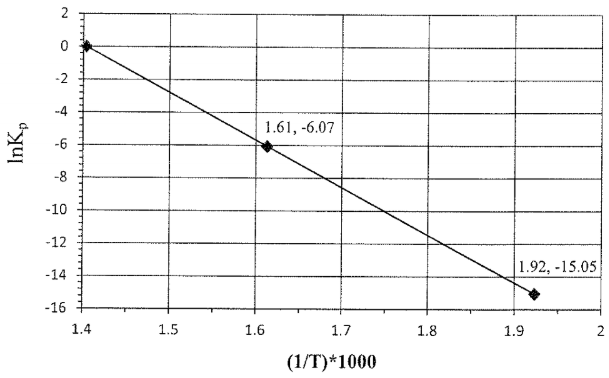# Problem: The temperature dependence of the equilibrium constant (Kp) is illustrated for the following reaction:NH4ClO4(s) → NH3(g) + HClO4(g)Note: the points are labeled as (1/T • 103, lnKp).Answer the following questions relating to the plot shown above:Under standard conditions, calculate the lowest temperature at which the reaction is spontaneous in the forward direction. Show all work and circle your final answer.

###### FREE Expert Solution

For this problem, we are being asked to calculate the lowest temperature at which the reaction is spontaneous in the forward direction

• In order for a reaction to be spontaneous or favor the forward reaction, Kp must be greater than 1 or ln(Kp) must be greater than 0
• In this case, we have to find the 1/T when ln(Kp) = 0
• Notice that this will be the y-intercept of the graph which can be seen as 1.4
99% (147 ratings)###### Problem Details

The temperature dependence of the equilibrium constant (Kp) is illustrated for the following reaction:

NH4ClO4(s) → NH3(g) + HClO4(g)

Note: the points are labeled as (1/T • 103, lnKp).Answer the following questions relating to the plot shown above:

Under standard conditions, calculate the lowest temperature at which the reaction is spontaneous in the forward direction. Show all work and circle your final answer.